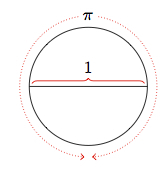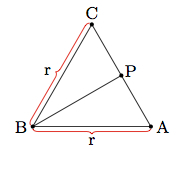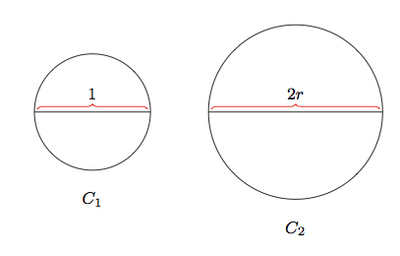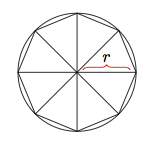# Circumference of a circle

Alignments to Content Standards: G-GMD.A.1

Suppose we define $\pi$ to be the circumference of a circle whose diameter is 1:Explain why the circumference of a circle with radius $r \gt 0$ is $2\pi r$.

## IM Commentary

The circumference of a circle of radius $r$ is $2\pi r$. This well known formula is taken up here from the point of view of similarity. It is important to note in this task that the definition of $\pi$ already involves the circumference of a circle, a particular circle. In order to show that the ratio of circumference to diameter does not depend on the size of the circle, a similarity argument is required. Two different approaches are provided, one using the fact that all circles are similar and a second using similar triangles. This former approach is simpler but the latter has the advantage of leading into an argument for calculating the area of a circle.

High school students will know that the circumference of a circle of radius $r$ is $2 \pi r$ and therefore the goal of this task is to help them understand this formula from the point of view of similarity. The first solution requires a general understanding of similarity of shapes while the second requires knowledge of similarity specific to triangles.

An alternative argument using trigonometric ratios provides a formula for the circumference of a regular polygon with $n \geq 3$ sides inscribed in a circle. A regular polygon with $n$ sides can be decomposed into $n$ isosceles triangles by drawing line segments connecting the center of the circle to the $n$ vertices of the polygon. An example of such a triangle (taken from a regular hexagon) is pictured below:As noted in the picture, these isosceles triangles have two sides of length $r$, the radius of the circle, and the third side $\overline{AC}$ has a length $b$ which depends on $m(\angle ABC)$. The point $P$ in the picture is the midpoint of $\overline{AC}$. So we have $\frac{|AP|}{|AB|} = \sin{\angle ABP}$ and from this we can deduce that $|AP| = r\sin{\angle ABP}$ and then, using that $P$ is the midpoint of $\overline{AC}$, $b = 2r\sin{\angle ABP}$. We know that 2n angles congruent to $\angle ABP$ make up a full circle so $m(\angle ABP) = \frac{360}{2n}$. The perimeter of the regular $n$ sided polygon inscribed in a circle is $n$ times the side length of this polygon, which we have just calculated: $$n \times 2r \sin{\left(\frac{360}{2n}\right)}.$$ Trigonometric ratios do not depend on the size of a triangle and so these formulas for $P_n$ allow us to deduce, as in Solution 2, that the ratio of the circumference of a circle to its diameter does not depend on the size of the circle. In addition, this provides a simple quantitative way to estimate $\pi$ by choosing a large value of $n$: of course this requires knowing the sine of an angle.

This first argument is an example of MP7, Look For and Make Use of Structure. The key to this argument is identifying that all circles are similar and then applying the meaning of similarity to the circumference. The second argument exemplifies MP8, Look For and Express Regularity in Repeated Reasoning. Here the key is to compare the circle to a more familiar shape, the triangle. A particularly efficient method is to divide a regular polygon approximating the circle into congruent triangles, making the formula for the perimeter and its relationship to $r$ particularly clear.

## Solutions

Solution: 1 Similarity of circles

Below is a picture of a circle of diameter 1, labelled $C_1$, and diameter $d = 2r$, labelled $C_2$:In the case pictured, $d$ is larger than $1$. All circles are similar and in this case the scale factor going from the circle of diameter $1$ to the circle of diameter $2r$ is $2r$. The circumference of a circle is a one dimensional measurement and so it scales in the same way as the diameters:

\begin{align} \frac{\text{Circumference}(C_2)}{\text{Circumference}(C_1)} &= \frac{\text{diameter}(C_2)}{\text{diameter}(C_1)} \\ &= \frac{2r}{1} \end{align}

Since the circumference of $C_1$ is $\pi$ by definition, it follows from the above equation that the circumference of $C_2$ is $2\pi r$.

Solution: 2 Similarity of triangles

In this solution we approximate the circumference of a circle using polygons and then use similarity of triangles to explain the formula for the circumference of a circle. Below is a picture of a regular octagon inscribed inside a circle of radius $r$:The circumference of the circle is a little bit more than the perimeter of the regular octagon which we can calculated using the picture below:The perimeter of the octagon is $8b$ since it has been divided into eight congruent triangles each with a base of $b$. We can calculate the angles of these eight triangles using the fact that the eight inner angles combine to make a 360 degree circle so each measures 45 degrees. The triangles are all isosceles so this means that the base angles each measure $\frac{180-45}{2} = 67.5$ degrees. By AAA, two triangles with angles $67.5^\circ, 67.5^\circ,$ and $45^\circ$ are similar. Therefore the ratio $(b:r)$ does not depend on the size of the regular octagon. This means that the ratio $(\text{perimeter(octagon)}:r)$ also does not depend on the size of the regular octagon. As we add more and more sides, this ratio approaches the ratio of the circumference of the circle to its radius. We conclude that for a circle $C$ of any radius $r$ $$(\text{circumference}(C):r) = \left(\pi:\frac{1}{2}\right).$$ Note the $\frac{1}{2}$ comes from looking at the circle of diameter 1 and circumference $\pi$: the radius of this circle is $\frac{1}{2}$. This is equivalent to the usual formula saying that the circumference of a circle with radius $r$ is $2\pi r$.